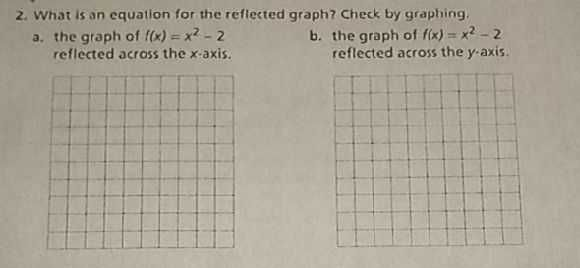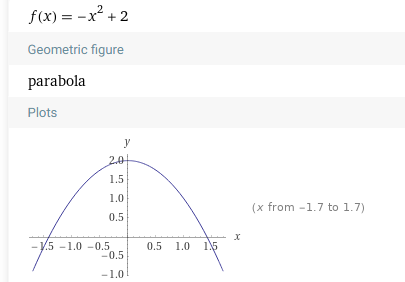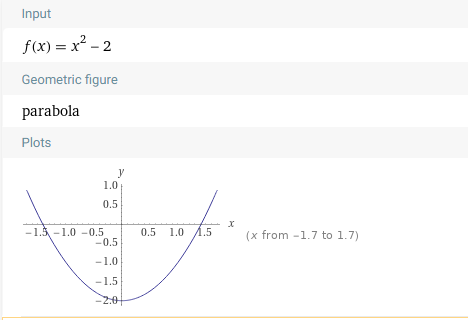### Still have math questions?

Algebra
Question2. What is an equation for the reflected graph? Check by graphing.

a. the graph of $$f ( x ) = x ^ { 2 } - 2$$

reflected across the $$x$$ -axis.

b. the graph of $$f ( x ) = x ^ { 2 } - 2$$

reflected across the $$y$$ -axis.